Subsections

# Ground state calculation

## quantum_groundstate

 extern double quantum_groundstate(quantum_reg *reg, double epsilon, quantum_reg H(MAX_UNSIGNED, double), int solver, double stepsize);

This function returns the ground state energy of the Hamiltonian implemented in the function H. The quantum register reg should have been initialized with quantum_new_qureg_size and filled with random amplitudes for best results. Upon return, reg will contain the ground state of the Hamiltonian. The desired absolute precision of the energy is controlled with epsilon. The variable solver allows to choose from different algorithms and can take any of the following values:

 Value Description QUANTUM_SOLVER_LANCZOS A straight-forward implementation of the Lanczos algorithm. As this implementation does not perform reorthogonalization, results may become unreliable for very large system sizes. QUANTUM_SOLVER_LANCZOS_MODIFIED A modified (and slower) version of the Lanczos algorithm [Dagotto, 1985] that is numerically stable. QUANTUM_SOLVER_IMAGINARY Imaginary time evolution using quantum_rk4.

Generally speaking, QUANTUM_SOLVER_LANCZOS is the fastest algorithm, but QUANTUM_SOLVER_LANCZOS_MODIFIED and QUANTUM_SOLVER_IMAGINARY are more reliable. Finally, the variable stepsize only needs to be supplied for QUANTUM_SOLVER_IMAGINARY and should correspond to the inverse of the typical energy scale of the Hamiltonian. Note that using the ground state solver will almost certainly require the use of double-precision arithmetic, which can be enabled by the --with-complex-type="double _Complex" configure switch.

## Example: Transverse Ising chain

The transverse Ising chain in a one-dimensional spinmodel is described by the Hamiltonian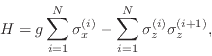where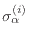refers to the Pauli spin matrix acting on theth spin,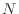is the total number of spins and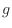is the magnitude of the external field in the transverse direction given in units of the ferromagnetic Ising interaction between the spins. Here, we are interested in periodic boundary conditions, i.e.,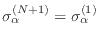. This Hamiltonian exhibits a quantum phase transition at the critical field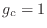from a ferromagnetic (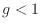) to a paramagnetic (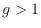) phase [Sachdev, 1999]. The ising demo program supplied with libquantum calculates the ground state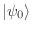and its spontaneous magnetization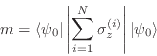for various values ofand different system sizes. From the theory of finite-size scaling [Cardy, 1996], it is known that close to the critical point,has to behave like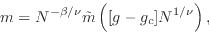where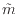is a rescaled magnetization function and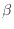and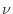are the critical exponents of the phase transition. Fig. 1 shows that plotting the magnetization data in a rescaled fashion causes all data points to collapse onto a single line. We can also use this data collapse to numerically extract values for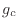and the critical exponents. Here, we find,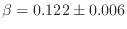, and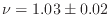, which is in very good agreement with the exact values of,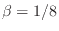, and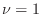.